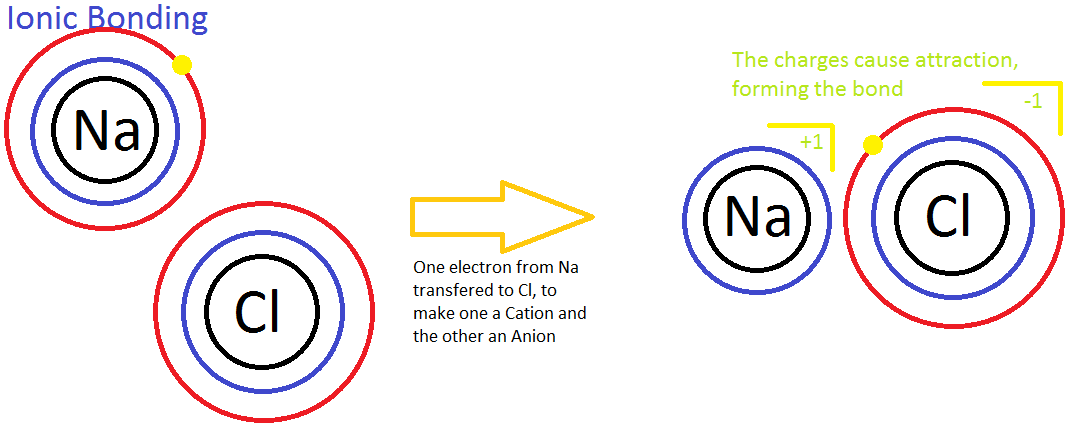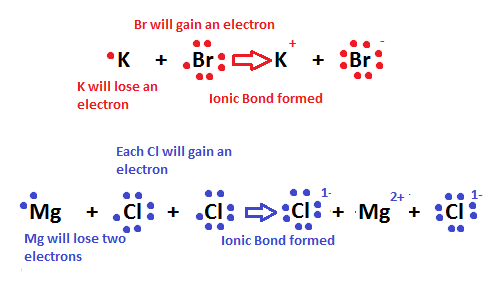# Introduction to Chemical Bonding

$$\newcommand{\vecs}{\overset { \rightharpoonup} {\mathbf{#1}} }$$ $$\newcommand{\vecd}{\overset{-\!-\!\rightharpoonup}{\vphantom{a}\smash {#1}}}$$$$\newcommand{\id}{\mathrm{id}}$$ $$\newcommand{\Span}{\mathrm{span}}$$ $$\newcommand{\kernel}{\mathrm{null}\,}$$ $$\newcommand{\range}{\mathrm{range}\,}$$ $$\newcommand{\RealPart}{\mathrm{Re}}$$ $$\newcommand{\ImaginaryPart}{\mathrm{Im}}$$ $$\newcommand{\Argument}{\mathrm{Arg}}$$ $$\newcommand{\norm}{\| #1 \|}$$ $$\newcommand{\inner}{\langle #1, #2 \rangle}$$ $$\newcommand{\Span}{\mathrm{span}}$$ $$\newcommand{\id}{\mathrm{id}}$$ $$\newcommand{\Span}{\mathrm{span}}$$ $$\newcommand{\kernel}{\mathrm{null}\,}$$ $$\newcommand{\range}{\mathrm{range}\,}$$ $$\newcommand{\RealPart}{\mathrm{Re}}$$ $$\newcommand{\ImaginaryPart}{\mathrm{Im}}$$ $$\newcommand{\Argument}{\mathrm{Arg}}$$ $$\newcommand{\norm}{\| #1 \|}$$ $$\newcommand{\inner}{\langle #1, #2 \rangle}$$ $$\newcommand{\Span}{\mathrm{span}}$$$$\newcommand{\AA}{\unicode[.8,0]{x212B}}$$

Chemical bonding is one of the most basic fundamentals of chemistry that explains other concepts such as molecules and reactions. Without it, scientists wouldn't be able to explain why atoms are attracted to each other or how products are formed after a chemical reaction has taken place. To understand the concept of bonding, one must first know the basics behind atomic structure.

## Introduction

A common atom contains a nucleus composed of protons and neutrons, with electrons in certain energy levels revolving around the nucleus. In this section, the main focus will be on these electrons. Elements are distinguishable from each other due to their "electron cloud," or the area where electrons move around the nucleus of an atom. Because each element has a distinct electron cloud, this determines their chemical properties as well as the extent of their reactivity (i.e. noble gases are inert/not reactive while alkaline metals are highly reactive). In chemical bonding, only valence electrons, electrons located in the orbitals of the outermost energy level (valence shell) of an element, are involved.

## Lewis Diagrams

Lewis diagrams are graphical representations of elements and their valence electrons. Valance electrons are the electrons that form the outermost shell of an atom. In a Lewis diagram of an element, the symbol of the element is written in the center and the valence electrons are drawn around it as dots. The position of the valence electrons drawn is unimportant. However, the general convention is to start from 12o'clock position and go clockwise direction to 3 o'clock, 6 o'clock, 9 o'clock, and back to 12 o'clock positions respectively. Generally the Roman numeral of the group corresponds with the number of valance electrons of the element.

Below is the periodic table representation of the number of valance electrons. The alkali metals of Group IA have one valance electron, the alkaline-earth metals of Group IIA have 2 valance electrons, Group IIIA has 3 valance electrons, and so on. The nonindicated transition metals, lanthanoids, and actinoids are more difficult in terms of distinguishing the number of valance electrons they have; however, this section only introduces bonding, hence they will not be covered in this unit.## Lewis diagrams for Molecular Compounds/Ions

To draw the lewis diagrams for molecular compounds or ions, follow these steps below (we will be using H2O as an example to follow):

1) Count the number of valance electrons of the molecular compound or ion. Remember, if there are two or more of the same element, then you have to double or multiply by however many atoms there are of the number of valance electrons. Follow the roman numeral group number to see the corresponding number of valance electrons there are for that element.

Valance electrons:

Oxygen (O)--Group VIA: therefore, there are 6 valance electrons

Hydrogen (H)--Group IA: therefore, there is 1 valance electron

NOTE: There are TWO hydrogen atoms, so multiply 1 valance electron X 2 atoms

Total: 6 + 2 = 8 valance electrons

2) If the molecule in question is an ion, remember to add or subract the respective number of electrons to the total from step 1.

For ions, if the ion has a negative charge (anion), add the corresponding number of electrons to the total number of electrons (i.e. if NO3- has a negative charge of 1-, then you add 1 extra electron to the total; 5 + 3(6)= 23 +1 = 24 total electrons). A - sign mean the molecule has an overall negative charge, so it must have this extra electron. This is because anions have a higher electron affinity (tendency to gain electrons). Most anions are composed of nonmetals, which have high electronegativity.

If the ion has a positive charge (cation), subtract the corresponding number of electrons to the total number of electrons (i.e. H3O+ has a positive charge of 1+, so you subtract 1 extra electron to the total; 6 + 1(3) = 9 - 1 = 8 total electrons). A + sign means the molecule has an overall positive charge, so it must be missing one electron. Cations are positive and have weaker electron affinity. They are mostly composed of metals; their atomic radii are larger than the nonmetals. This consequently means that shielding is increased, and electrons have less tendency to be attracted to the "shielded" nucleus.

From our example, water is a neutral molecule, therefore no electrons need to be added or subtracted from the total.

3) Write out the symbols of the elements, making sure all atoms are accounted for (i.e. H2O, write out O and 2 H's on either side of the oxygen). Start by adding single bonds (1 pair of electrons) to all possible atoms while making sure they follow the octet rule (with the exceptions of the duet rule and other elements mentioned above).

4) If there are any leftover electrons, then add them to the central atom of the molecule (i.e. XeF4 has 4 extra electrons after being distributed, so the 4 extra electrons are given to Xe: like so. Finally, rearrange the electron pairs into double or triple bonds if possible.## Octet Rule

Most elements follow the octet rule in chemical bonding, which means that an element should have contact to eight valence electrons in a bond or exactly fill up its valence shell. Having eight electrons total ensures that the atom is stable. This is the reason why noble gases, a valence electron shell of 8 electrons, are chemically inert; they are already stable and tend to not need the transfer of electrons when bonding with another atom in order to be stable. On the other hand, alkali metals have a valance electron shell of one electron. Since they want to complete the octet rule they often simply lose one electron. This makes them quite reactive because they can easily donate this electron to other elements. This explains the highly reactive properties of the Group IA elements.

Some elements that are exceptions to the octet rule include Aluminum(Al), Phosphorus(P), Sulfur(S), and Xenon(Xe).

Hydrogen(H) and Helium(He) follow the duet rule since their valence shell only allows two electrons. There are no exceptions to the duet rule; hydrogen and helium will always hold a maximum of two electrons.

## Ionic Bonding

Ionic bonding is the process of not sharing electrons between two atoms. It occurs between a nonmetal and a metal. Ionic bonding is also known as the process in which electrons are "transferred" to one another because the two atoms have different levels of electron affinity. In the picture below, a sodium (Na) ion and a chlorine (Cl) ion are being combined through ionic bonding. Na+ has less electronegativity due to a large atomic radius and essentially does not want the electron it has. This will easily allow the more electronegative chlorine atom to gain the electron to complete its 3rd energy level. Throughout this process, the transfer of the electron releases energy to the atmosphere.Another example of ionic bonding is the crystal lattice structure shown above. The ions are arranged in such a way that shows unifomity and stablity; a physical characteristic in crystals and solids. Moreover, in a concept called "the sea of electrons," it is seen that the molecular structure of metals is composed of stabilized positive ions (cations) and "free-flowing" electrons that weave in-between the cations. This attributes to the metal property of conductivity; the flowing electrons allow the electric current to pass through them. In addition, this explains why strong electrolytes are good conductors. Ionic bonds are easily broken by water because the polarity of the water molecules shield the anions from attracting the cations. Therefore, the ionic compounds dissociate easily in water, and the metallic properties of the compound allow conductivity of the solution.## Covalent Bonding

Covalent bonding is the process of sharing of electrons between two atoms. The bonds are typically between a nonmetal and a nonmetal. Since their electronegativities are all within the high range, the electrons are attracted and pulled by both atom's nuceli. In the case of two identical atoms that are bonded to each other (also known as a nonpolar bond, explained later below), they both emit the same force of pull on the electrons, thus there is equal attraction between the two atoms (i.e. oxygen gas, or O2, have an equal distribution of electron affinity. This makes covalent bonds harder to break.There are three types of covalent bonds: single, double, and triple bonds. A single bond is composed of 2 bonded electrons. Naturally, a double bond has 4 electrons, and a triple bond has 6 bonded electrons. Because a triple bond will have more strength in electron affinity than a single bond, the attraction to the positively charged nucleus is increased, meaning that the distance from the nucleus to the electrons is less. Simply put, the more bonds or the greater the bond strength, the shorter the bond length will be. In other words:

Bond length: triple bond < double bond < single bond

## Polar Covalent Bonding

Polar covalent bonding is the process of unequal sharing of electrons. It is considered the middle ground between ionic bonding and covalent bonding. It happens due to the differing electronegativity values of the two atoms. Because of this, the more electronegative atom will attract and have a stronger pulling force on the electrons. Thus, the electrons will spend more time around this atom.The symbols above indicate that on the flourine side it is slightly negitive and the hydrogen side is slightly positive.

## Polar and Non-polar molecules

Polarity is the competing forces between two atoms for the electrons. It is also known as the polar covalent bond. A molecule is polar when the electrons are attracted to a more electronegative atom due to its greater electron affinity. A nonpolar molecule is a bond between two identical atoms. They are the ideal example of a covalent bond. Some examples are nitrogen gas (N2), oxygen gas (O2), and hydrogen gas (H2).One way to figure out what type of bond a molecule has is by determining the difference of the electronegativity values of the molecules.

If the difference is between 0.0-0.3, then the molecule has a non-polar bond.

If the difference is between 0.3-1.7, then the molecule has a polar bond.

If the difference is 1.7 or more, then the molecule has an ionic bond.